Jazz Theory 9 THE OVERTONE SERIES
Rhythm Class - In Focus - Learn to Read Music - Jazclass Links

(

JT 9.1 - The Overtone Series

Many of the basic principles and harmonic developments of music originate from the acoustic make up of a single tone.

When a length of string (or column of air) is made to vibrate it produces the basic pitch of this string, called the Fundamental.
Let us look at a low C string (on a piano for example.)

1. The full wavelength produces a low C = the Fundamental No.1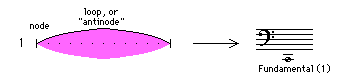2. Simultaneously this same string also vibrates at a wavelength half of the fundamental wavelength.

Half the wavelength produces a low C, 1 octave higher = Overtone No.2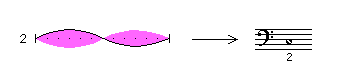3. The string also vibrates at a third of the fundamental wavelength.

One third of the wavelength produces a G, a 5th higher = Overtone No.3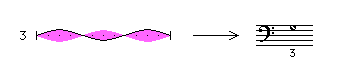4. The same string also vibrates simultaneously at a quarter of the fundamental wavelength.

One quarter of the wavelength produces a c, a 4th higher = Overtone No.4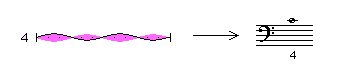The same string also vibrates at wavelengths of :

5. a fifth of the Fundamental wavelength = Overtone No.5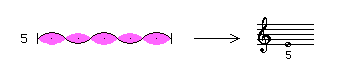6. a sixth of the Fundamental wavelength = Overtone No.67. a seventh of the Fundamental wavelength = Overtone No.7

8. an eighth of the Fundamental wavelength = Overtone No.8

and so on.

An infinite number of vibrations, dividing the fundamental string into an ever increasing number of equal wavelengths.

Each of these vibrations produces their own pitch.
This composite of sounds accompanies the Fundamental tone and is called the Overtone Series.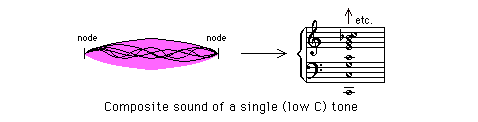(Down - Up - Top - Links)

JT 9.2 - Some Characteristics

Here is the Overtone Series up to the 16th Overtone.
They all sound at the same time of course, but I have spread them out over the length of the staff. This provides a good overview of all members of the Series.
I have also played them in ascending order on the Audio.

Audio 1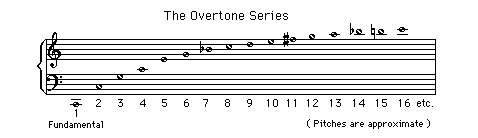Here are some useful characteristics of the Overtone Series you should know :

1. the intervals between successive Overtones gradually becomes smaller.
Chord voicings that simulate the structure of the overtone series, with larger intervals at the bottom and smaller intervals at the top, will have a clearer and more resonant sound.

2. the Number of each Overtone expresses its wavelength as a fraction of the fundamental wavelength :

• Overtone No.3 = 1/3 of the Fundamental wavelength

• Overtone No.5 = 1/5 of the Fundamental wavelength

• Overtone No.16 = 1/16 of the Fundamental wavelength

• Overtone No.89 = 1/89 of the Fundamental wavelength

Overtones are therefore also called partials.

3. the Fundamental tone is repeated each octave, and its Overtone No. increases each time by a magnitude of 2.
For the overtones of C' for example :

• No. 1 = C' (the Fundamental)
• No. 2 = 2 x 1 = C
• No. 4 = 2 x 2 = c
• No. 8 = 2 x 4 = c'
• No.16 = 2 x 8 = c"
• No.32 = 2 x 16 = c"'
• No.64 = 2 x 32 = c""
etc.

4. the number of Overtones doubles within each successive octave.

5. the first 8 pitches of the Overtone Series, expressed in scale tone numbers of the major scale are :

Audio 2(Down - Up - Top - Links)

JT 9.3 - What the Ear perceives

The human ear (or rather the human brain) consciously perceives in most cases only the fundamental pitch of the tone.
This is partly because all overtones have a nodal point (point of no vibration) at the nodal points of the fundamental and thus reinforce the fundamental tone.Another important reason why we only tend to distinguish the fundamental tone is that three of the first four tones of the Overtone series represent the same (fundamental) tone at successive octave levels :The human brain recognises the composite of overtones as the characteristic sound and timbre of an instrument.
For the vibration intensity of individual overtones varies considerably for each type of instrument.

This is largely how we recognise the difference in sound between a piano, a flute, a guitar, a saxophone, and so on.

(Down - Up - Top - Links)

JT 9.4 - Experiments

Experiment 1

1. Open the lid of an acoustic piano so that you can reach the strings inside with your hand.

2. Now place your foot on the sustain pedal and play the lowest C on the keyboard.

3. Stop the vibration of the C string with a finger but leave your foot on the sustain pedal.

4. The sound you now hear are the vibrations of other strings which have absorbed the various overtone vibrations from the low C string.

The vibrations will slowly fade, lower overtones first.

Experiment 2
Do the same experiment with a C one octave higher.

Dampen the string with your finger again . You can now still hear the fundamental pitch of this string, for it vibrates as Overtone No.2 on the C string an octave below.

(Down - Up - Top - Links)

JT 9.5 - The Perfect Intervals

Note that the first three intervals of the overtone series are the perfect intervals.

Audio 3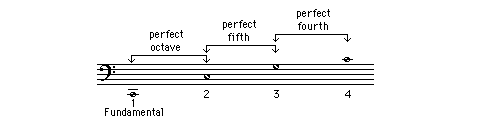The first step towards harmony in Western music, significantly, started with these intervals.

It commenced in the 9th Century, when the single melody line of the traditional plainsong was doubled in parallel octaves, fifths and fourths to form the first organum.
This allowed basses, tenors, altos and sopranos to sing the melody together, each within their own vocal range.

The intervals of the octave and the perfect fifth have ever since remained the two most important building blocks in Western music.

• The octave providing the framework of stability and repetition.

• The fifth generating the primary harmonic driving force, the Dominant to Tonic cadence.
(The Tonic is the first note, the Dominant the fifth note of a major scale)

(
Down - Up - Top - Links)

JT 9.6 - Grandparent - Parent - Child

We can compare the Overtone Series to a family.

The Fundamental is the head of this family of tones :the Parent (C below). The other tones represent various off springs.

The Oldest Child in this comparison is the first different tone (from a C) : Overtone No. 3 (the G below).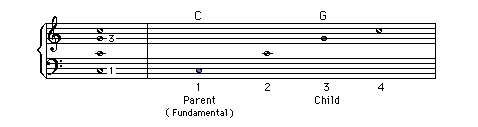If we look at the same comparison in the Overtone Series for the Fundamental F :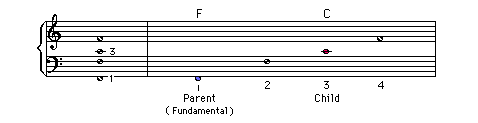If C is the Parent of G, but also the Child of F we see the emergence of three successive generations :

Child

Parent

Grandparent

G

C

F

If we look at the Overtone Series of Bb we find the 'oldest child' to be (an octave plus) a perfect fifth up, namely F.

Bb in its turn, is the 'child' of Eb, and so on.

Audio 4

Child

Parent

Grandparent

G

C

F

C

F

Bb

F

Bb

Eb

Bb

Eb

Ab

In this way we can identify the acoustic relationships than include all 12 tones of our musical System. More about this in the next Lesson.

(
Down - Up - Top - Links)

JT 9.7 - Quiz

A.
What are the pitches of the Overtones 2 to 8 for the Fundamental G ?

B.
What are the pitches of the Overtones 2 to 8 for the Fundamental D ?

C.
What are the pitches of the Overtones 2 to 8 for the Fundamental A ?

D.
Given a Low B fundamental produced on a 1280 mm long string.
How long is the wavelength of Overtone No.256, and what is its pitch ?

E.
Complete the Child - Parent - Grandparent Diagram above until all 12 notes of our musical system are included.

(Down - Up - Top - Links)

JT 9.8 - Lesson Material

File Name Contents
jt09fac.gif Jazz Theory 9 - Facts sheet
jtx101.gif Keyboard Diagrams

Manuscript paper